RPL+ for RPL Users  (MorphEngine v.1.4.1)In addition to RPL as found on the HP-50g calculator, ND1 supports RPL+.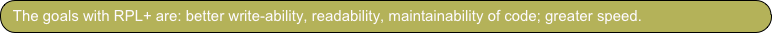RPL+ is a superset of RPL. It extends the RPL language, where every new feature is mix- and matchable: you can use it in stead of equivalent (but more complicated, slower performing) RPL statements, but you don’t have to.

This document provides a complete list of new features in RPL+ and provides a few RPL-to-RPL+ conversion examples. Read this if you already know RPL and want to know what’s new.

If you don’t know RPL, read Learning RPL+ instead of this text. If you want a quick overall view on the language, or if you’re coming from another language and want to see how RPL+ fits in, see the RPL+ Quick Reference.

RPL+ extensions are primarily in the following areas:

1. -locals; easier definition and re-assignment, easier incrementing and basic operations, via new fused = operators

2. -arrays (and lists); easier indexing and assignment/adding, and a hash-table feature, via new fused [] operator

3. -unification of lists and vectors; under the array umbrella, they’re the same now. This opens many new use possibilities with existing commands

4. -immediate algebraic expressions; algebraic expressions may appear as non-decorated in-fix notation anywhere; such expressions are evaluated immediately; this has a few limits but permits in-fix notation right in RPL

5. -command set; not directly part of the language (and therefore not discussed here but found in the Function Reference) but close to the language, there’re many new array and array processing commands that greatly impact the expressiveness of the language: filter, select, find, doUntil, combine, etc. Various of them take program fragments (in program, array, or string form), just like RPL’s DOSUBS, STREAM, etc. family of commands (which is also supported).

RPL code that uses much array access or locals is typically sped up by at least a factor of two, by adopting simple RPL+ code changes. RPL code that fully adopts RPL+ is often written much more compactly and can be 10x faster.

There’re various downloadable folders that contain RPL+ code. A lot of examples can be found in Project Euler.

A running log of future RPL+ features is available as a forum topic.

Overview

Program/Command Execution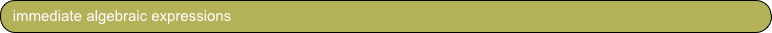Undecorated expressions are allowed and evaluated automatically.

expr

‘expr‘ EVAL

There must be no space characters in expr.

Examples:

1 3*n-5 FOR

1 ‘3*n-5’ EVAL FOR     or the equivalent     1 3 n * 5 - FOR

x>=5-3

x 5 3 - >=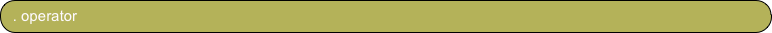folder.program permits function/command/program program to be called in the folder named folder

If the folder name consists of multiple words, use a concatenation of the initials of each word instead.

For example, to access the function phi in folder Number Theory, write NT.phi

Local Variables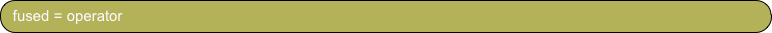Define/assign, and re-define/re-assign at any time, a local variable.

=x

→ x ≪ ... ≫

or any subsequent

'x' STO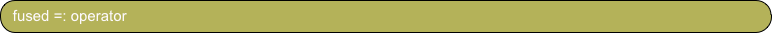Like = without consuming the top-of-stack item.

=:x

→ x ≪ x ... ≫

or any subsequent

'x' STO x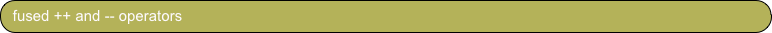Type-aware local variable increment and decrement.

++x

'x' 1. STO+     or      'x' 1 STO+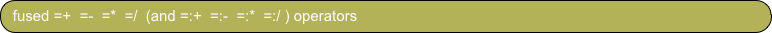Type-aware, argument-based local variable increment, decrement, multiply, or divide, with option to not consume top-of-stack item.

=+x                =:+x

'x' STO+       DUP ‘x’ STO+

=-x                =:-x

'x' STO-        DUP ‘x’ STO-

=*x                =:*x

'x' STO*        DUP ‘x’ STO*

=/x                =:/x

'x' STO/        DUP ‘x’ STO/

Array access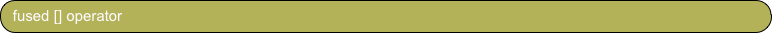One- and zero-based array read access.

x[expr]

'x' commands to implement expr GET    or the equivalent     'x(expr)' EVAL

For example, for expr equals j-k

x[j-k]

'x' j k - GET    or the equivalent     'x(j-k)' EVAL

x[j-k:0]

'x' j k - 1 + GET    or the equivalent     'x(j-k+1)' EVAL

Indexes wrap, permitting circular buffers.

For (j-k) > length of array x:

x[j-k]

'x' j k - x SIZE LIST→ MOD 1 + GET    or the equivalent     'x(MOD(j-k, [sizeOfX])+1)' EVAL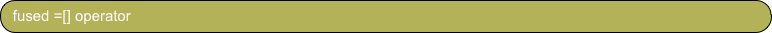One- and zero-based array write access.

=x[expr]

'x' commands to implement expr PUT    or the equivalent     'x(expr)' STO

For example, for expr equals j-k

=x[j-k]

'x' j k - PUT    or the equivalent     'x(j-k)' STO

=x[j-k:0]

'x' j k - 1 + PUT    or the equivalent     'x(j-k+1)' STO

For indices j > length of array x, an error is thrown, except the element following the last can be assigned.

For example, for x equals [1 2 3]

=x

'x' SWAP +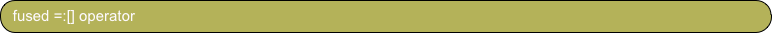Like =[] without consuming top-of-stack item:

=:x[j-k]

DUP 'x' j k - PUT    or the equivalent     DUP 'x(j-k)' STO

and

=:x[j-k:0]

DUP 'x' j k - 1 + PUT    or the equivalent     DUP 'x(j-k+1)' STO

and, for j == length of array x:

=:x[j]

DUP 'x' SWAP +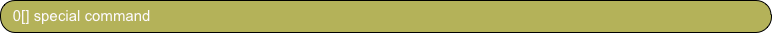"Default to zero-based indexing" command.

Permits to drop any ":0" in subsequent array accesses. If one-based indexing is desired, specify ":1" in array access.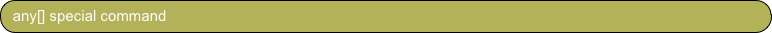"Enable any index" command.

Permits you to use any numerical index, or string, or other object, to be used as index when using array accessors. This disables out-of-bounds detection, implies zero-based indexing, and enables sparse arrays and hash-tables (dictionaries).

Others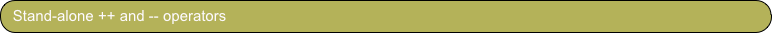Type-aware top-of-stack element increment and decrement.

++

1. +     or      1 +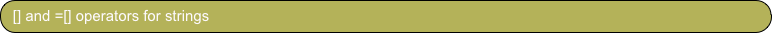Allow access to characters in strings, and work just like those for arrays. Both one- and zero-based indexing are supported.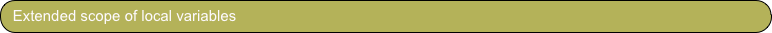Inner-frame (called) RPL code has access to the local variables of outer-frame (calling) RPL code.

Inner-frame local variable definitions supersede outer-frame definitions of the same name.

Among other uses, this feature enables anonymous local functions, as in:

{DUP {SWAP OVER MOD Euclid EVAL} {DROP} IFTE} =Euclid Euclid EVAL ≫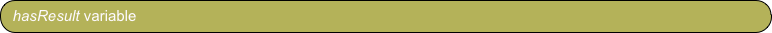Global boolean variable set by find, match, DOSUBS, DOLIST, SEQ indicating whether operation yielded new items on the stack.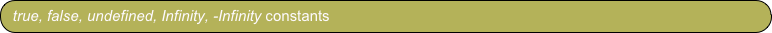New constants. true and false can be entered and are returned by comparison operators. Infinity and -Infinity can be entered and are returned by certain functions for certain input values.

undefined can occur in special situations and when using sparse arrays.

For example,

any[] [] =a “zero” =a “three” =a a ≫   yields   [“zero” (undefined) (undefined) “three”]

RPL+ Language Extensions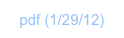The following shows the usage of the local variable and array access language features in RPL+ and provides a change-by-change account of the speed-ups obtained.

The example code below can be downloaded to ND1, via the shared folder “RPL+”.

RPL+ program:

=n

0 'n>295' {R→I} IFT =zero

n mkpents =pents

[0 1 1 0] =signs

{1} =partitions

0[] @ select zero-based array indexing

1 n FOR j

zero @ running sum

1 =k

WHILE

j pents[k] - =:diff

0 >=

REPEAT

partitions[diff]

signs[k] {+} {-} IFTE

++k

END

=partitions[j]

NEXT

partitions[n]

The equivalent RPL program:

1: ≪ → n ≪
2:   0. 'n>295' {R→I} IFT → zero ≪
3:   0. → k ≪
4:   n mkpents → pents ≪
5:   [0. 1. 1. 0.] → signs ≪
6:   {1.} → partitions ≪
7:   1. n FOR j
8:      zero @ running sum
9:      1. 'k' STO
10:      WHILE
11:         j pents k GET -
12:         DUP @ for re-use next
13:         0. >=
14:      REPEAT
15:         partitions SWAP 1 + GET
16:         signs k 4. MOD 1. + GET {+} {-} IFTE
17:         'k' 1. STO+
18:      END
19:      DROP
20:      'partitions' SWAP STO+ @ add to partitions
21:   NEXT
22:   partitions n 1. + GET
23: ≫≫≫≫≫≫≫

Pretending the RPL program would have been our starting-point, here are the steps to arrive at the RPL+ version:

First goal is to replace all STOs into arrays with RPL+ array write syntax:

Step 1: Use array write syntax to append values to partitions array
Replace
20:      'partitions' SWAP STO+ @ add to partitions
with
20:      =partitions[j]
Speed gain: 150%

The next goal is to replace all GETs with array read syntax:

Step 2: Replace obvious array access
Replace
11:         j pents k GET -
with
11:         j pents[k] -
Speed gain: 22%

Step 3: Use local variable to hold re-used intermediate result instead of stack
Replace
12:         DUP @ for re-use next
15:         partitions SWAP 1 + GET
19:      DROP
with
11:         j pents[k] - =:diff
15:         partitions diff 1 + GET
(diff is assigned the difference of j and pents[k] and does not affect the stack)
Speed gain: 1% (little, but we now have a local variable, diff, to use as array index in the next step)

Step 4: Replace line 15 with zero-based array access
15:         partitions diff 1 + GET
becomes
15:         partitions[diff:0]
We can drop the ":0" syntax (which stands for "zero-based indexing"), if we change the default mode for array indexing from one-based to zero-based via
6.5:        0[]
Line 15 can now be written:
15:         partitions[diff]
Speed gain: 20%

Step 5: Replace access in line 16
16:         signs k 4. MOD 1. + GET {+} {-} IFTE
by
16:         signs[MOD(k,4)] {+} {-} IFTE
Speed gain: 50%

Step 6: RPL+ automatically supports circular buffers.

Line 16 can more simply be written
16:         signs[k] {+} {-} IFTE
Speed gain: 3%

Step 7: Use local increment syntax
Replace
17:         'k' 1. STO+
with
17:         ++k
Speed gain: 20%

The rest of the changes do not affect the speed as they're outside the inner loops, but they do enhance readability:

Step 8: Replace other read array accesses
22:   partitions n 1. + GET
becomes
22:   partitions[n]

Step 9: Use = operator instead of → ≪ and subsequent STOs into locals

These two lines
3:   0. → k ≪
9:      1. 'k' STO
become
9:      1 =k
In RPL+ there's no need to open a new defining procedure when you need a local variable. You can create one at any time and use the same '=' syntax to assign and re-assign values.

Similarly,
1: → n ≪
4: → pents ≪

5: → signs ≪

6: → partitions ≪

become
=n

=pents

=signs

=partitions

Total speed gain: 500%

(The Real version of this same algorithm has a gain of 300%, with the one write array access producing a smaller gain, and the other changes producing relative larger gains.)

Example: “Partitions of an Integer”

Following extensive testing in this discussion thread, this RPL code is the most efficient for converting a string into a list of its character codes:

DUP SIZE

-> s

≪

1 s START

DUP NUM SWAP TAIL

NEXT

DROP s ->LIST

≫

Here’s RPL+ code to accomplish the same:

=s

{} =list

1 s SIZE FOR i

s[i] NUM =list[i]

NEXT

list

Speed gain: 200%

Adopting a specific instruction in MorphEngine (the calculator’s engine), split, which breaks a string (or array, or number) into its parts, the following RPL+ code can be written:

split ≪ NUM ≫ MAP ≫

Speed gain: 210%

Further exploiting automatic array processing in RPL+:

split NUM ≫    or (using “modern” syntax)     split charCode ≫

Speed gain: 350%

Example: “String to list of character codes”

RPL for this popular benchmark problem:

8 0 0 0 0 → R S X Y A

≪

1 R START 0 NEXT R →ARRY 'A' STO

DO

X 1 + 'X' STO

A X R PUT 'A' STO

DO

S 1 + 'S' STO

X 'Y' STO

WHILE Y 1 > REPEAT

Y 1 - 'Y' STO

A X GET A Y GET -

IF DUP 0 == SWAP ABS X Y - == OR THEN

0 'Y' STO

A X A X GET 1 - PUT 'A' STO

WHILE A X GET 0 == REPEAT

X 1 - 'X' STO

A X A X GET 1 - PUT 'A' STO

END

END

END

UNTIL Y 1 == END

UNTIL X R == END

S

≫

After straight adoption of RPL+ features:

8 =r

0 =:s =:x =y

[r] 0 CON =a

DO

++x

r =a[x]

DO

++s

x =y

WHILE y 1 > REPEAT

--y

a[x] a[y] - =:diff

IF diff 0 == SWAP ABS x y - == OR THEN

0 =y

a[x] -- =a[x]

WHILE a[x] 0 ==

REPEAT

--x

a[x] -- =a[x]

END

END

END

UNTIL y 1 == END

UNTIL x r == END

s

Speed gain: 150%

Using more RPL+ features, incl. immediate algebraic expressions, yields this code:

8 =r

0 =:s =:x =y

[] =a

DO

r =a[++x]

DO

++s

x =y

WHILE y>1 REPEAT

a[x] a[--y] - =t

IF OR(t==0,x-y==abs(t)) THEN

0 =y

WHILE a[x] -- =:a[x] 0 ==

REPEAT

--x

END

END

END

UNTIL y==1 END

UNTIL x==r END

s

Speed gain: >200%

Example: “Eight Queens Problem”

Here’s the final example from Learning RPL+:

1000 primes                    @ generate primes under 1000

[ 100 > ] filter DUP          @ filter for three-digit numbers; duplicate result

[ phi ] [ 1 == ] doUntil      @ iterate the Euler totient until the result is 1

NIP                           @ drop the result array of final values

[ 10 == ] select              @ select the primes which were iterated 10x

head                          @ return the first number

Speed gain: ?

This program finds the first three-digit prime, that, when iterated with Euler’s totient function (which computes the number of co-primes of a number), yields an iteration chain length of 10.

Change head to size and get a count of all three-digit primes that meet that condition. Remove the last statement altogether, and see all the matching primes.

RPL+ continues the push into functional programming that the HP-48 line started when it begun to offer STREAM, DOSUBS, MAP, and SEQ functions. (All of which are also in ND1.)

It would be hard to rewrite the problem above in RPL. It would certainly be a lot longer and the expectation is that the comparative speed-up obtained by using these functional array processing functions would be significant.

(That’s because these functions are not only more legible than control structures, they also perform largely in native space, where processing is faster.)

Example: “Primes producing a certain totient chain length”